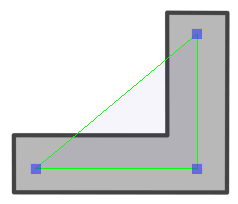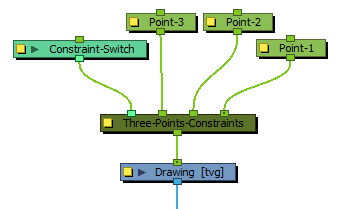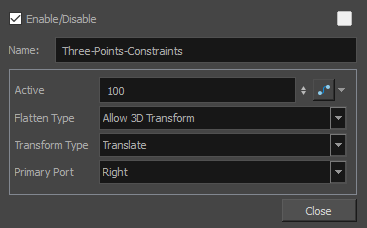# Three-Points Constraint Node

The Three-Points Constraint translates, rotates and scales a drawing to make it follow the pivots of the three pegs connected to it.## Connection

The Three-Points Constraint node must be connected to three Peg nodes. The pivot point of each peg will define the resting position of one of the points used to transform the drawing connected under it. When one of the pegs is moved, the Three-Points Constraint will transform the drawing so that it follows the pivot point of that peg.NOTE The resting position of the points is based on the pivot point of the peg. The pivot point of a peg can be set by selecting the peg with the Translate, Rotate, Scaleor Skewtool, then clicking and dragging the pivot in the Camera view, or by entering its coordinates in the layer properties of the peg.

Optionally, the leftmost input port of a Three-Points Constraint node can be connected to a Constraint-Switch, which can be used to reduce the effect of the Three-Points Constraint node on the element it is connected by setting its Active parameter between 0 and 100.

## Controls

When the controls of the Three-Points Constraint are displayed, the position of the three points are displayed with blue squares linked by a green line.

These points are only a visual representation and cannot be manipulated.

## PropertiesParameter Description Active The percentage of influence the constraint has on its child element. If set to 100, the element will follow the three points closely. If set to 0, the element will not be affected by the constraint. If a Transformation Switch node is connected to the Three-Points Constraint node, the Active parameters of the Transformation Switch and the Three-Points Constraint nodes will be multiplied together. Flatten Type This parameter defines what happens if one of the pegs defining the constraint points is moved on the Z-axis: Ignore 3D Transform: The element will be constrained to the vertical and horizontal position of the pegs, and will ignore their position on the Z axis. Allow 3D Transform: The element will be 3D-rotated to follow the position of the pegs in the 3D space. Project to Plane: The element will be constrained in 2D, but will be made to follow the perceived position of the points from the camera's point of view. This means the 3D position of the points will be projected on a 2D plane that is parallel to the camera. NOTE This projection is based on the position of the primary point peg on the Z-axis. Hence, if the three pegs are on a different position on the Z-axis, there may be a perceived offset between the position of the non-primary points and their effect on the element. Transform Type By default, only the position of the points is used to transform the element. Since those points are defined by pegs, these pegs can also be subjected to scale, angle and skew transformations that would be normally ignored by the Three-Points Constraint node. This parameter can be used to apply the other transformations done on the peg of the primary point on the element, rather than ignoring them. Translate: Ignores the transformations done on the primary peg, only applies the transformations required for the element to track the three points. Transform: Applies the transformations calculated by the Three-Points Constraint node to make the element to track the three points, then applies the scale, angle and skew values of the primary peg on top of them. Local Transform: Applies the scale, angle and skew values of the primary peg on the element, then applies the transformations calculated by the Three-Points Constraint node for the element to track the three points. Primary Port Defines which one of the ports, and hence, which one of the pegs the node is connected to, is used to define the primary point. The primary point affects the other parameters in the following ways: When the Flatten Type is set to Ignore 3D Transform or Project to Plane, the position of the element on the Z-axis will be the one of the primary point. When the Flatten Type is set to Project to Plane, the position of the primary point on the Z-axis is used as the plane on which to project the 3D positions of the points. If the Transform Type is set to Transform or Local Transform, the scale, angle and skew transformations of the primary peg will be applied to the element.